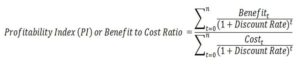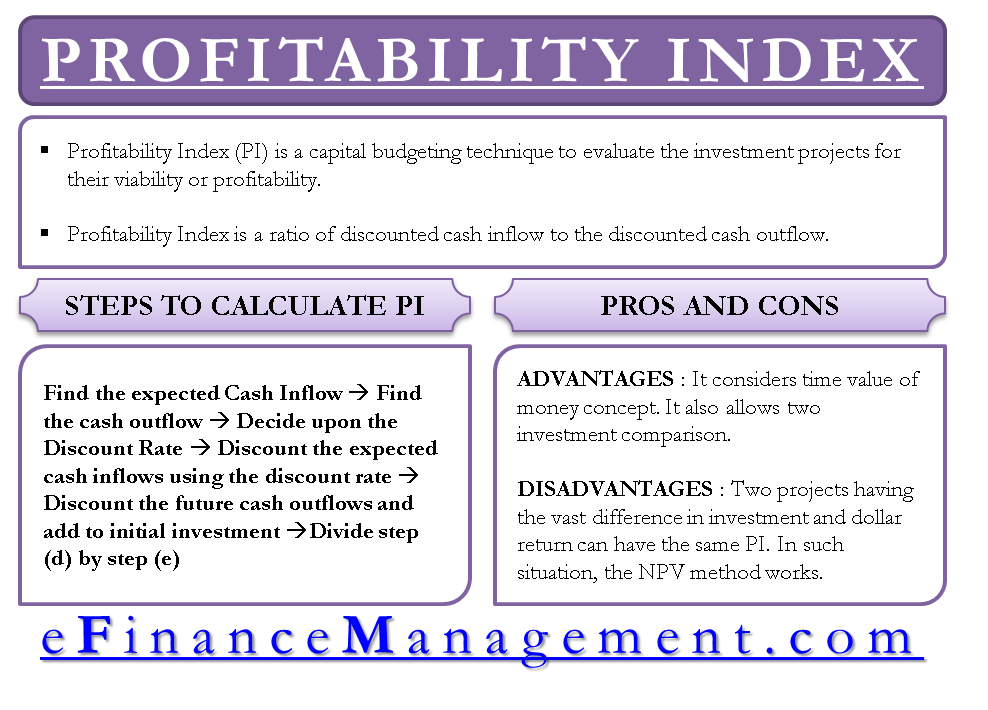# Profitability Index (PI) or Benefit-Cost Ratio

Profitability Index (PI) is a capital budgeting technique to evaluate the investment projects for their viability or profitability. Discounted cash flow technique is used in arriving at the profitability index. It is also known as a benefit-cost ratio. Calculation of profitability index is possible with a simple formula with inputs as – discount rate, cash inflows, and outflows. PI greater than or equal to 1 is interpreted as a good and acceptable criterion.

## Profitability Index Definition

Profitability Index is a ratio of discounted cash inflow to the discounted cash outflow. Discounted cash inflow is our benefit in the project and the initial investment is our cost, which is why we also call it benefit to cost ratio.

### Profitability Index Method

The method used for arriving at profitability index of a proposed project is explained stepwise below:a)     Find the expected cash inflows of the project

b)    Find the cash outflows of the project (Initial Investment + any other cash outflow)

c)     Decide an appropriate discount rate

d)    Discount the expected cash inflows using the discount rate

e)     Discount the future cash outflows and add to initial investment

f)     Divide step (d) by step (e)

### How to calculate the Profitability Index

The calculation of PI is easily possible once we have the cash inflows and outflows with appropriate discount rate are in place.

### Profitability Index Formula

The formula indicates the benefits in the numerator and costs in the denominator. The formula for calculating Profitability Index is as follows:### Example of Profitability Index (PI)

Let’s assume the cash flows of a project as mentioned year wise in the second column of the below table. The negative cash flows are the costs and positive ones are the benefits. In the third column, they are discounted at 10% rate. All the discounted benefits are added to make \$ 16,832 and discounted costs to make \$15,450.

 Year Cash Flows Discounted Benefits Costs (CF) CF @ 10% 0 -10000 -10000 10000 1 5000 4545 4545 2 5000 4132 4132 3 -5000 -3757 3757 4 4000 2732 2732 5 4000 2484 2484 6 -3000 -1693 1693 7 3000 1539 1539 8 3000 1400 1400 Total 1382 16832 15450 NPV 16832/15450= 1.09 Profitability Index

The benefit to cost ratio or the PI can be found out by dividing benefits by costs (16832/15450 = 1.382)### Acceptance Criteria or Interpretation

A profitability index of anything equal to or greater than 1 is considered good. It means that the project is worth executing. PI greater than 1 indicates that the project is paying something more than the required rate of return of the investor. In our example, the project should be accepted as it is greater than 1 i.e. 1.09.

### Profitability Index (PI) and Net Present Value (NPV)

Profitability Index is closely linked with net present value. Both will present same results as far as acceptance and rejection are concerned. It is because the almost same calculation is followed in both. In PI, we divide our benefits by our costs whereas, in NPV, we deduct our costs from the benefits. PI will give a relative value and contrarily

## Profitability Index – Advantages and Disadvantages

The advantage of profitability method is that it considers the time value of money and presents a relative profitability of the project. Relative profitability allows comparison of two investments irrespective of their amount of investment. A higher PI would indicate a better IRR and a lower PI would have lower IRR.

The main disadvantage of the PI method is also its relative indications. Two projects having the vast difference in investment and dollar return can have the same PI. In such situation, therefore, the NPV method remains the best method.

Last updated on : May 13th, 2019
What’s your view on this? Share it in comments below.

#### 5 Comments

1.Imedi
2.Boluwatife
3.Isiaka
•Sanjay Bulaki Borad